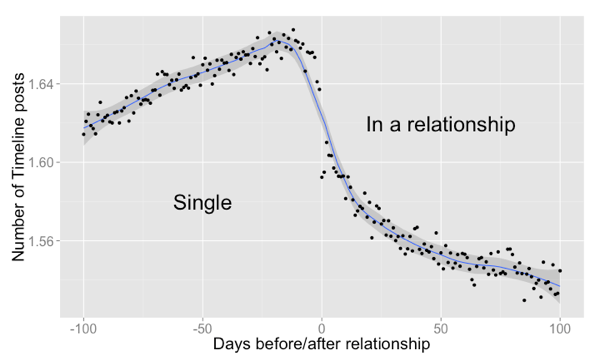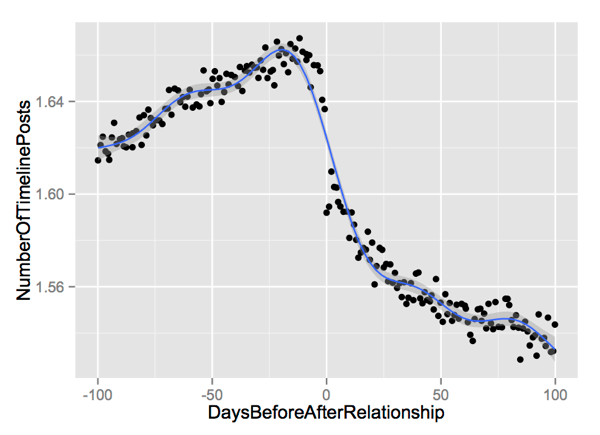# The gap between data mining and predictive models

The Facebook data science blog shared some fun data explorations this Valentine’s Day in Carlos Greg Diuk’s “The Formation of Love”. They are rightly receiving positive interest in and positive reviews of their work (for example Robinson Meyer’s Atlantic article). The finding is also a great opportunity to discuss the gap between cool data mining results and usable predictive models. Data mining results like this (and the infamous “Beer and Diapers story”) face an expectation that one is immediately ready to implement something like what is claimed in: “Target Figured Out A Teen Girl Was Pregnant Before Her Father Did” once an association is plotted.

Producing a revenue improving predictive model is much harder than mining an interesting association. And this is what we will discuss here.

The following very interesting graph is shared in “The Formation of Love”:The data preparation is not explained in great detail (that is okay, this is popular blog post- not a formal paper requiring a material and methods section). But it looks like the y-axis of the graph is something like directed timeline posts per day, the x-axis is day number (relative to when a relationship is declared), and each dot represents a person or a group of people (more on this last point in a bit). The point of the graph is: there is an increase in timeline posts between people who end up declaring a relationship on Facebook and then a drop off after the declaration of relationship. This is interesting behavioral data. The unwarranted expectation is with such a strong relation you could use immediately use daily posting rates to identify who is likely to declare a relationship and when this might happen (this would be valuable as these are unobserved until “day 0”).

However, as many other commenters have noticed: the range of posts per day is very small ranging from 1.62 well before declaration, to 1.65 right before declaration and settling to 1.54 well after declaration. And the variation away from the smoothing line seems unbelievable low (the fit is way too good). Finally the dots are not grouped at integer rates or rates with small denominators (suggesting each dot is in fact already aggregated from many users, and it is likely a jitter has been added to make the plot more legible). The possibility of aggregation is consistent with the start of the article which says: “This research has been conducted on anonymized, aggregated data.” The question is: how much aggregation is going on? Trends that can be seen in large aggregates are often very hard to apply to individuals. Or alternately very weak individual effects can be made to stand out if you aggregate enough data. There is no question in my mind we have a valid statistically significant result in front of us- it is just is there enough practical or “clinical significance” to immediately drive usable individual predictions?

To start our investigation let’s get an approximate copy of the data from the graph. This can be done by running the graph through an online digitizing tool such as: WebPlotDigitizer. A little work yields the data file NMsg.csv. And a small bit of R code lets us reproduce the graph.

``` # load libraries require(RCurl) require(ggplot2) require(mgcv) require(reshape2) # Load data, and make sure x is increasing urlBase <- 'https://raw2.github.com/WinVector/Examples/master/MiningGap/' mkCon <- function(nm) { textConnection(getURL(paste(urlBase,nm,sep=''))) } d <- read.table(mkCon('NMsg.csv'),header=T,sep=',') d <- d[order(d\$DaysBeforeAfterRelationship),] # plot something similar to original graph ggplot(data=d,aes(x=DaysBeforeAfterRelationship, y=NumberOfTimelinePosts)) + geom_point() + stat_smooth(method = "gam", formula = y ~ s(x)) ```

Which yields a graph fairly similar to the original:We generated our intensity estimates as follows:

``` earlyIntensity <- mean( d[d\$DaysBeforeAfterRelationship >= -100 & d\$DaysBeforeAfterRelationship < -80, 'NumberOfTimelinePosts']) print(earlyIntensity) ##  1.622637 nearIntensity <- mean( d[d\$DaysBeforeAfterRelationship >= -20 & d\$DaysBeforeAfterRelationship < 0, 'NumberOfTimelinePosts']) print(nearIntensity) ##  1.653082 afterIntensity <- mean( d[d\$DaysBeforeAfterRelationship >= 80 & d\$DaysBeforeAfterRelationship < 100, 'NumberOfTimelinePosts']) print(afterIntensity) ##  1.539452 ```

And here is the problem for a predictive model. If we assume (without justification) that users are generating these messages with from a Poisson model with the above given intensities we find it would be difficult to tell from the number of posts if a user is 100 days from declaring a relationship, about to declare one, or long after declaring one. Now the Poisson assumption is unjustified (and whole families of methods, such as over-dispersed models, have been developed to work around its limits) but it is a reasonable first guess for any sort of event counts per interval problem.

Below is a bar-chart of the probability distribution (under our Poisson model with the estimated intensities) of the observed number of posts expected from a person right before they declare a relationship compared to a person long after they declared a relationship (the pair of situations most easy to tell apart by posting rates):The issue is: the two distributions are almost indistinguishable. We can’t tell how many posts a person would make given their group (long time from declaration, about to declare, or long after declaration), therefore we can’t reliably tell what group a person is in given their post count (a sort of contrapositive version of Bayes’ law).

The code to make the bar chart is as follows:

``` # What would individuals generating posts # as a Poisson process with a given intensity # look like around 100 days before forming # a relationship, right before forming a relationship, # and long after a relationship is formed? density <- data.frame(NumberOfTimelinePosts=0:10) density\$nearCounts <- dpois( density\$NumberOfTimelinePosts, lambda=nearIntensity) density\$afterCounts <- dpois( density\$NumberOfTimelinePosts, lambda=afterIntensity) dm <- melt(density, id.vars=c('NumberOfTimelinePosts'), variable.name='group', value.name='density') ggplot(data=dm) + geom_bar(stat='identity',position='dodge', aes(x=NumberOfTimelinePosts,y=density,fill=group)) + scale_x_continuous(breaks=density\$NumberOfTimelinePosts) ```

But our bar chart is ignoring even larger blockers to making a usable predictive model. 100 days before a person declares a relationship we would not know how many days they are away from declaring (we know after they declare how many days ago they declared, but we don’t know the future) and we don’t know a priori who they are thinking of declaring with. A business doesn’t want a system that uses things that are hard to know (who will get into a relationship with whom) to predict easy measurements (post counts) after the fact. A business wants a real time system that uses things that are easy to measure (post counts) to predict future events that are valuable (for example: who will declare a relationship with whom and when). The original graph shows a relation between things, but a business needs a directional inference (from what is easy and cheap to things that are valuable).

And this is the jump: a plotted relation is not always immediately the basis for a usable predictive model. The popular press and management do not always seem to remember this distinction.

We started the article guessing that the dots must be aggregations of users (otherwise such tight error bars would be beyond what could be expected). Let’s use our (unjustified) Poisson process assumption to estimate how many users are likely aggregated in each dot. To attempt this we need to appeal to three facts:

1. The mean equals the variance for a Poisson distribution.
2. For independent identically distributed data the expected square distance between pairs of points is twice the variance.
3. When you average or aggregate k independently identically distributed items this average has an expected variance of 1/k of the original items.

For individuals emitting posts in a Poisson distribution of intensity 1.5 posts per day we would expect a variance of 1.5 yielding error bars of around +-1.2, much larger than on the graphs we have seen. So we expect each dot is the aggregation of k individuals for some large k.

Suppose x is a random variable that is the average of k independent identically distributed Poisson distributed random variables (each with intensity lambda). Then we expect: E[x]/Var[x] to be independent of lambda and approximately equal to k (we are a little disturbed that our estimate is not dimensionless, but we must remember that Poisson distributions of different intensities have different shapes). Under this reasoning we estimate each dot on the graph represents almost 70,000 individuals. Under our (unjustified) Poisson assumption somebody with less data than this could not get an aggregate graph as tight as the one presented (at such low activity rates, however it is possible that aggregating data across many days could in fact give usable predictive models).

This illustrates the large gap between a good data mining result and a profitable predictive model.

The R-code to estimate the de-trended variance and k (the degree of aggregation) is a as follows:

``` # If the original data was generated from # independent individuals with slowly varying # Poisson intensity, then we could estimate the # degree of aggregation to see the mean/variance # ration in the plot. odd <- 2*(1:(dim(d)[]/2))-1 varEst <- sum((d[odd,'NumberOfTimelinePosts'] -d[odd+1,'NumberOfTimelinePosts'])^2)/(2*length(odd)) print(varEst) ##  2.289848e-05 meanEst <- mean(d\$NumberOfTimelinePosts) print(meanEst) ##  1.599492 kEst <- meanEst/varEst print(kEst) ##  69851.47 print(dim(d)[]) ##  193 ```

(Note: Corrected an error on my part. I used to try and estimate the total study size multiplying the number of dots times the estimated number of subjects per dot. That made no sense as each dot is presumably the same set of individuals- so the total study size is estimated at around 70,000 people, not 13 million.)

More Win-Vector LLC work on post-hoc inspection of results include:### jmount

Data Scientist and trainer at Win Vector LLC. One of the authors of Practical Data Science with R.

### 3 replies ›

1.Tal Galili says:

Great post, thank you :)

2.Bob says:

This particular line of code does not appear to work.
ggplot(data=dm) +
geom_bar(stat=’identity’,position=’dodge’,
aes(x=NumberOfTimelinePosts,y=density,fill=group)) +
scale_x_continuous(breaks=density\$NumberOfTimelinePosts)

I get:
Don’t know how to automatically pick scale for object of type data.frame. Defaulting to continuous

I’m new to R. Is there something missing or does running this require a certain version of R or ggplot?

3.jmount says:

@Bob Thanks for the feedback and sorry you are having trouble. The first guesses of what might be going wrong are: I made a mistake, different software versions, bad cut/paste, or variables changed value as I worked and the plot doesn’t work until later in the session (another possible error on my part).

On the cut/paste issue: in your comment (and likely in my article) the single quotes have been promoted up to some sort of pretty apostrophe thing that R does not understand (so I can’t paste the code from your comment and get it to work). The way around that is to use code from the original R file (which I just double checked does run, a mistake on my part is an un-mentioned third possibility): https://github.com/WinVector/Examples/blob/master/MiningGap/plot.R .

On the software version: issue ggplot2 has gone through a number of changes that do change the API (but if you are new to R I would hope you are up to date). To find out what version of everything is loaded try the sessionInfo() command. Here is what I get:

``` > sessionInfo() R version 3.0.2 (2013-09-25) Platform: x86_64-apple-darwin10.8.0 (64-bit) locale:  en_US.UTF-8/en_US.UTF-8/en_US.UTF-8/C/en_US.UTF-8/en_US.UTF-8 attached base packages:  stats graphics grDevices utils datasets methods base other attached packages:  reshape2_1.2.2 mgcv_1.7-26 nlme_3.1-111 ggplot2_0.9.3.1 RCurl_1.95-4.1  bitops_1.0-6 loaded via a namespace (and not attached):  colorspace_1.2-4 dichromat_2.0-0 digest_0.6.4 grid_3.0.2  gtable_0.1.2 labeling_0.2 lattice_0.20-23 MASS_7.3-29  Matrix_1.0-14 munsell_0.4.2 plyr_1.8 proto_0.3-10  RColorBrewer_1.0-5 scales_0.2.3 stringr_0.6.2 tools_3.0.2 ```

I also re-installed RCurl, ggplot2, mgcv and reshape2 and the plot still worked for me.

On the other issues: I re-ran the whole script from a clean workspace (after adding explicit prints to the ggplot commands to force the graphs to be produced even when there is not implicit print).

So the code “should” work, sorry you are having trouble.